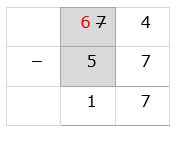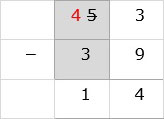# Subtraction of Two 2-digit Numbers With Borrowing

Advertisements

Subtract the numbers 74 − 57

### Solution

Step 1:

We line up the numbers vertically.Step 2:

In the ones column, we cannot subtract 7 from 4 as 7 is greater than 4. So we borrow a ten from tens column. We strike off 7 and write a 6 and put a 1 beside 4 to make it 14. Now we subtract 14 – 7 = 7. 7 is written down below ones column.Step 3:

Next in the tens column, we subtract 6 − 5 = 1. 1 is written down below tens column. So the answer is 17.Subtract 53 − 39

### Solution

Step 1:

Align the numbers vertically.Step 2:

In the ones column, we cannot subtract 9 from 3 as 9 is greater than 3. So we borrow a ten from 5 in the tens column. We strike off 5 and write a 4 to its left. We put a 1 to left of 3 to make it 13. (1 ten = 10 ones). Now we 13 − 9 = 4. 4 is written below ones column.Step 3:

Next in the tens column, 4 – 3 = 1. 1 is then written below tens column and the final answer is 14.Advertisements